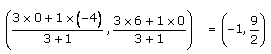# RD Sharma Solutions for Class 10 Maths Chapter 14- Co-ordinate Geometry Exercise 14.3

Division of a line segment can be even done by marking a point on the line segment. This exercise majorly deals with problems based on section formulae. The RD Sharma Solutions Class 10 is the right tool to learn the correct steps of solving such problems. Students wishing to build confidence in solving this exercise can also download the RD Sharma Solutions for Class 10 Maths Chapter 14 Co-ordinate Geometry Exercise 14.3, PDF provided below.

## RD Sharma Solutions for Class 10 Maths Chapter 14 Co-ordinate Geometry Exercise 14.3 Download PDF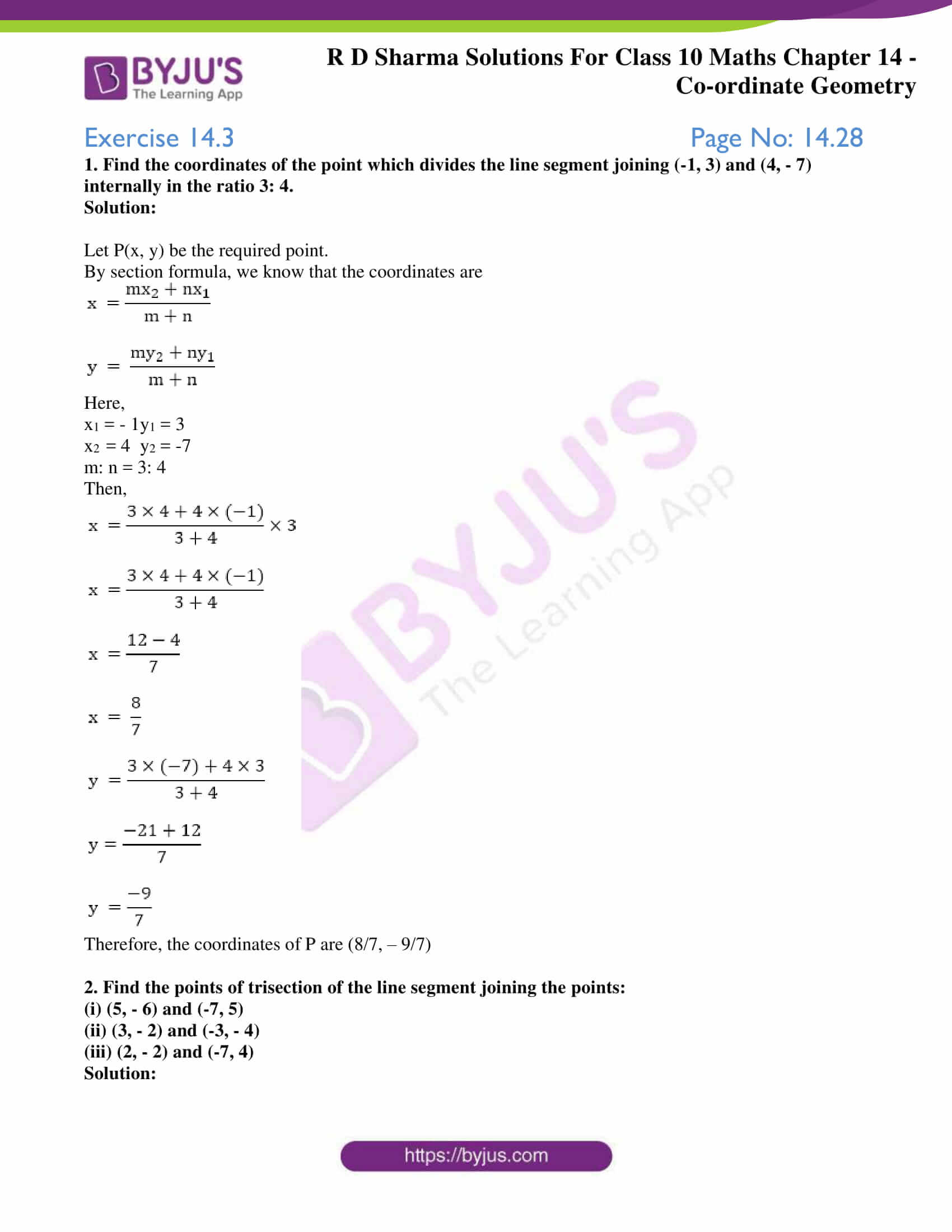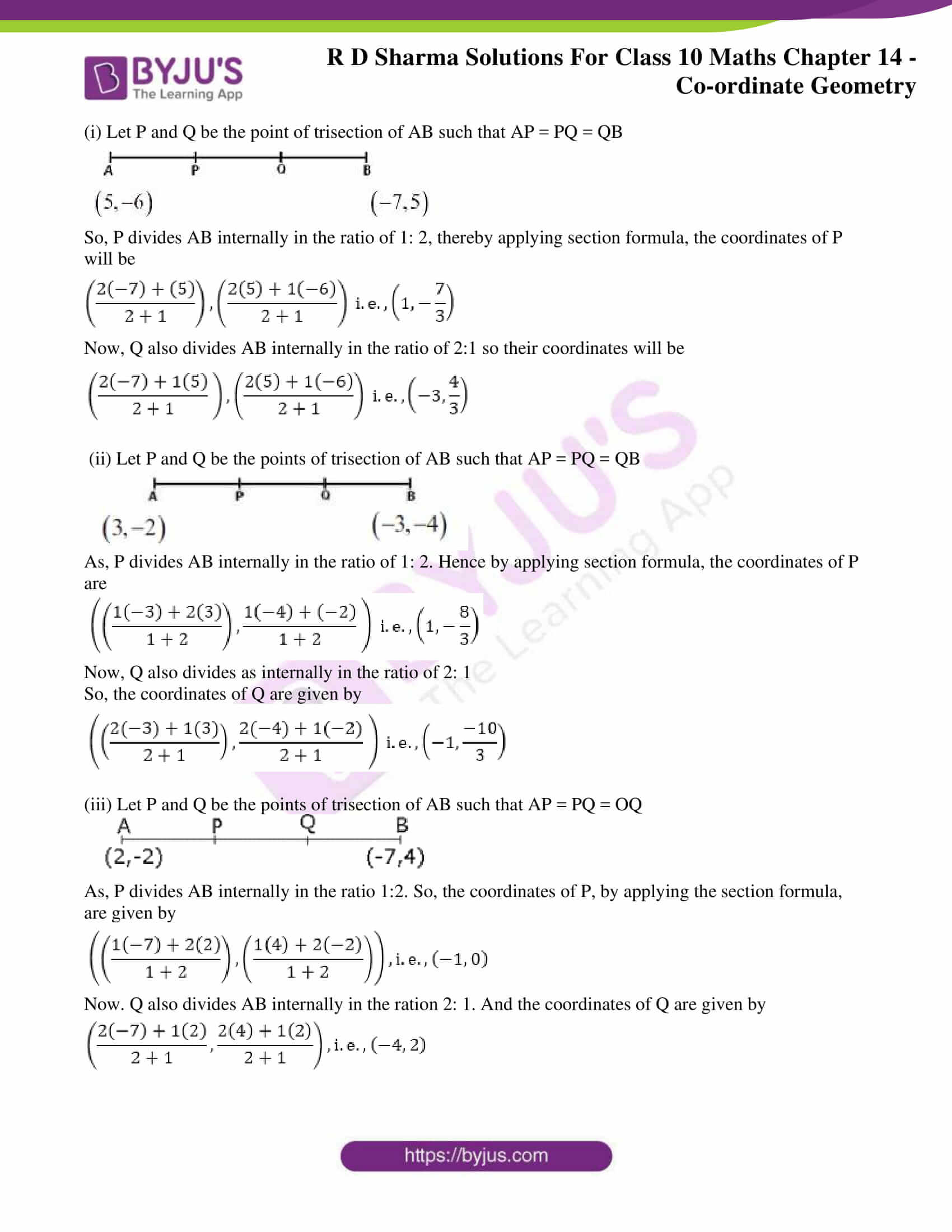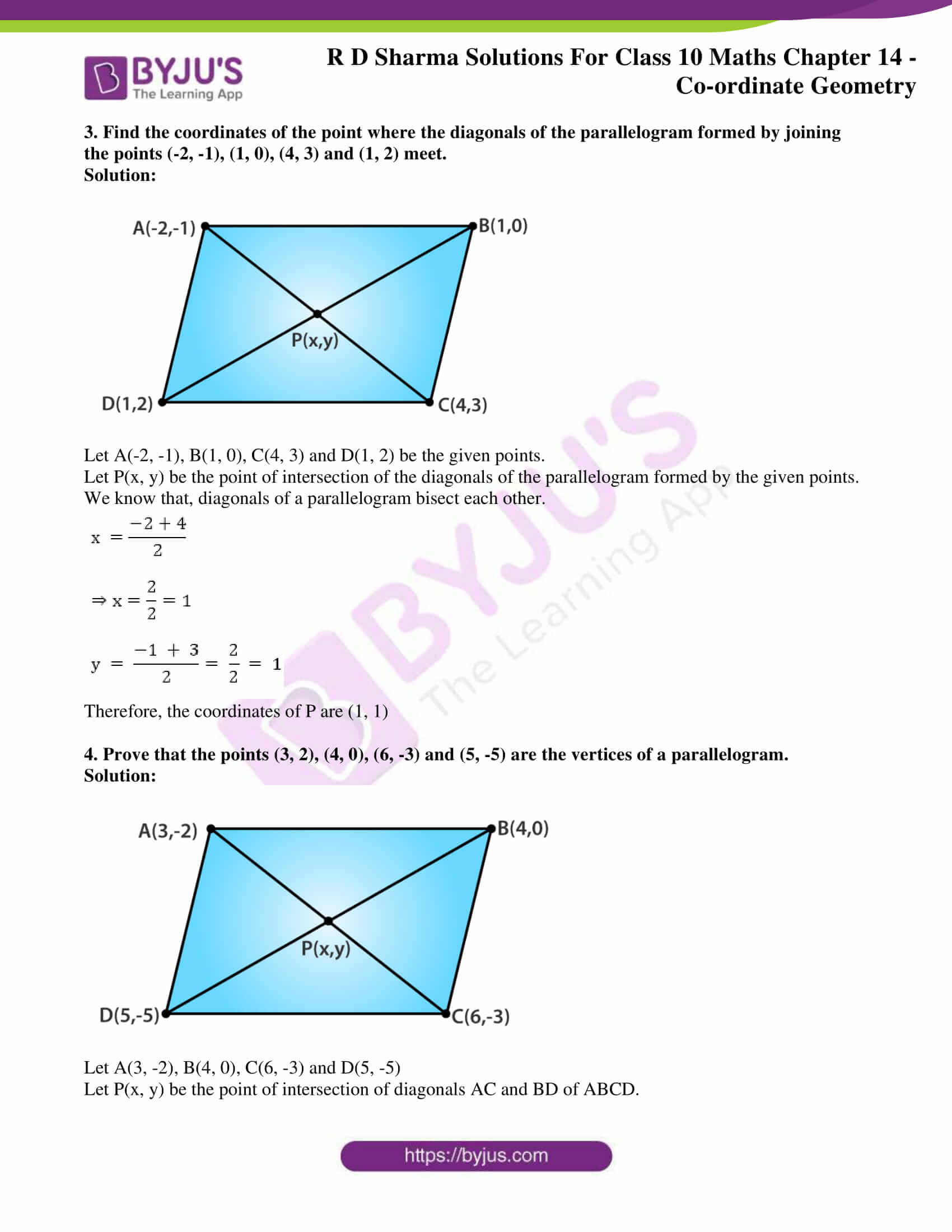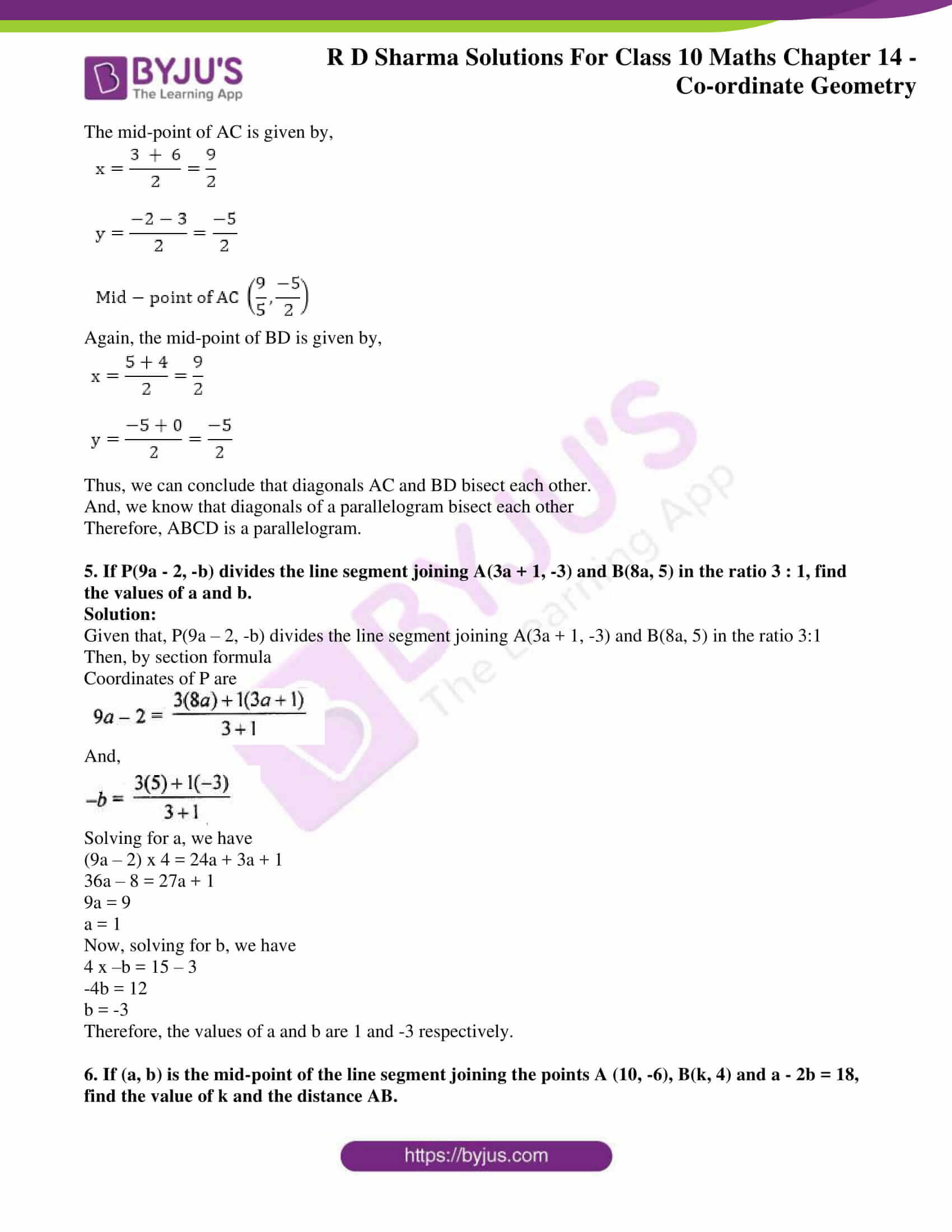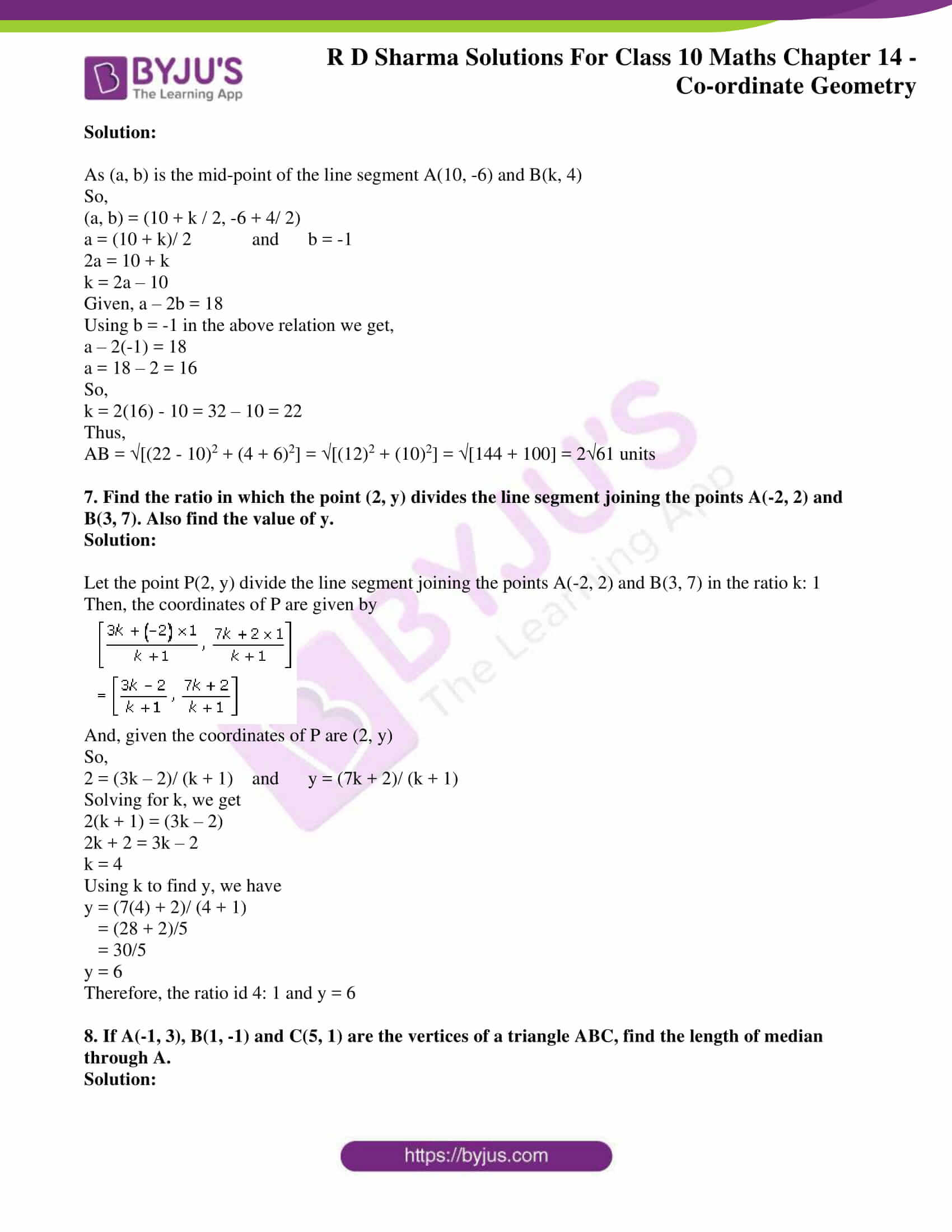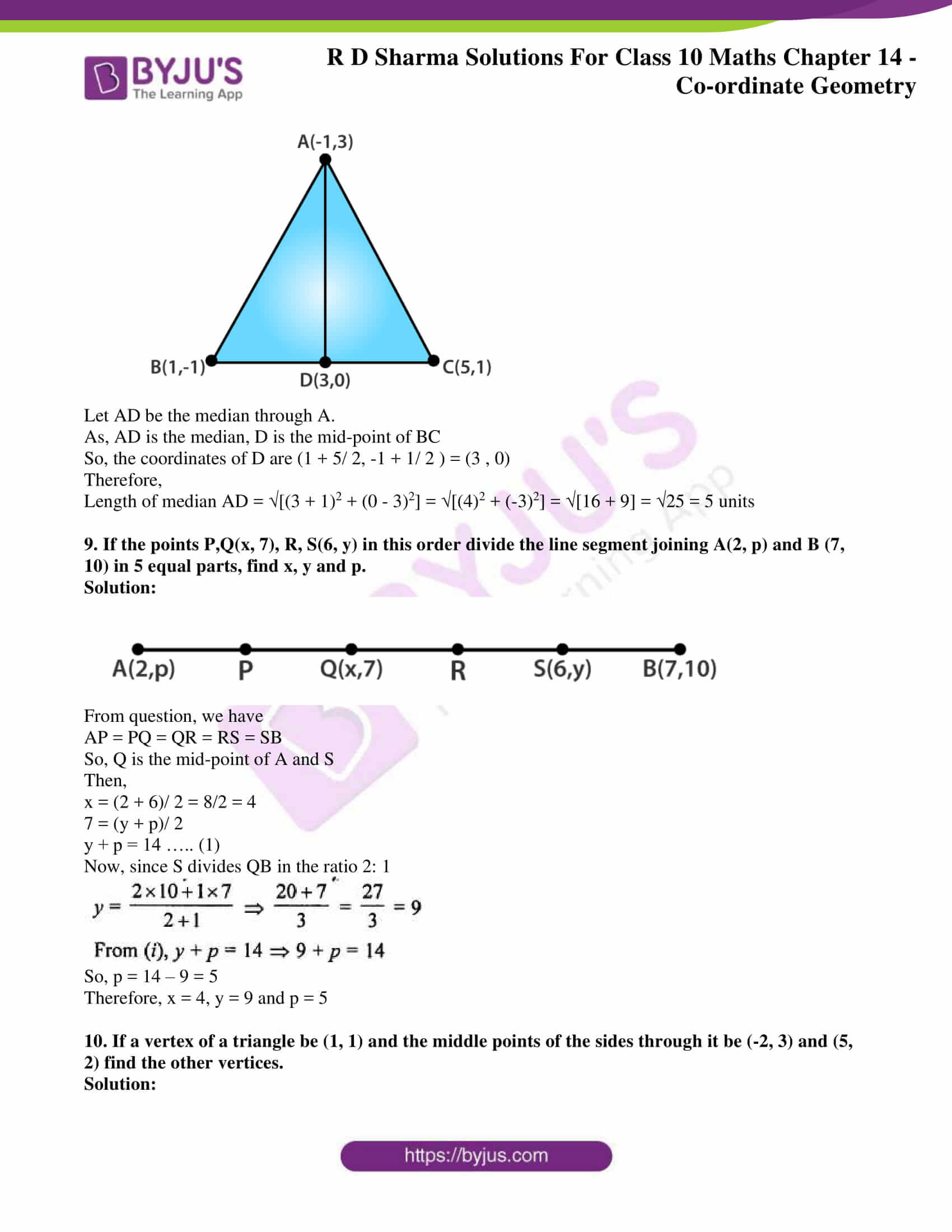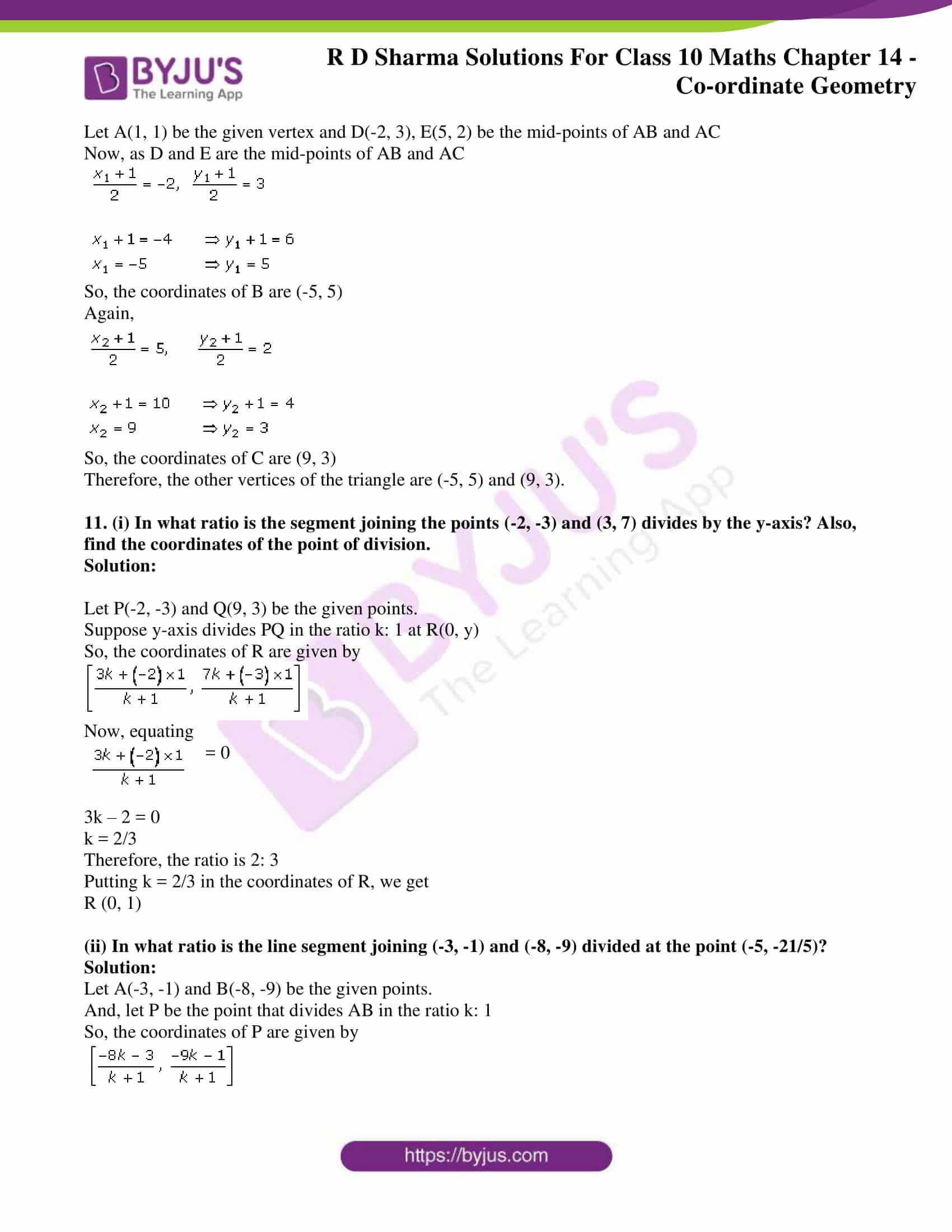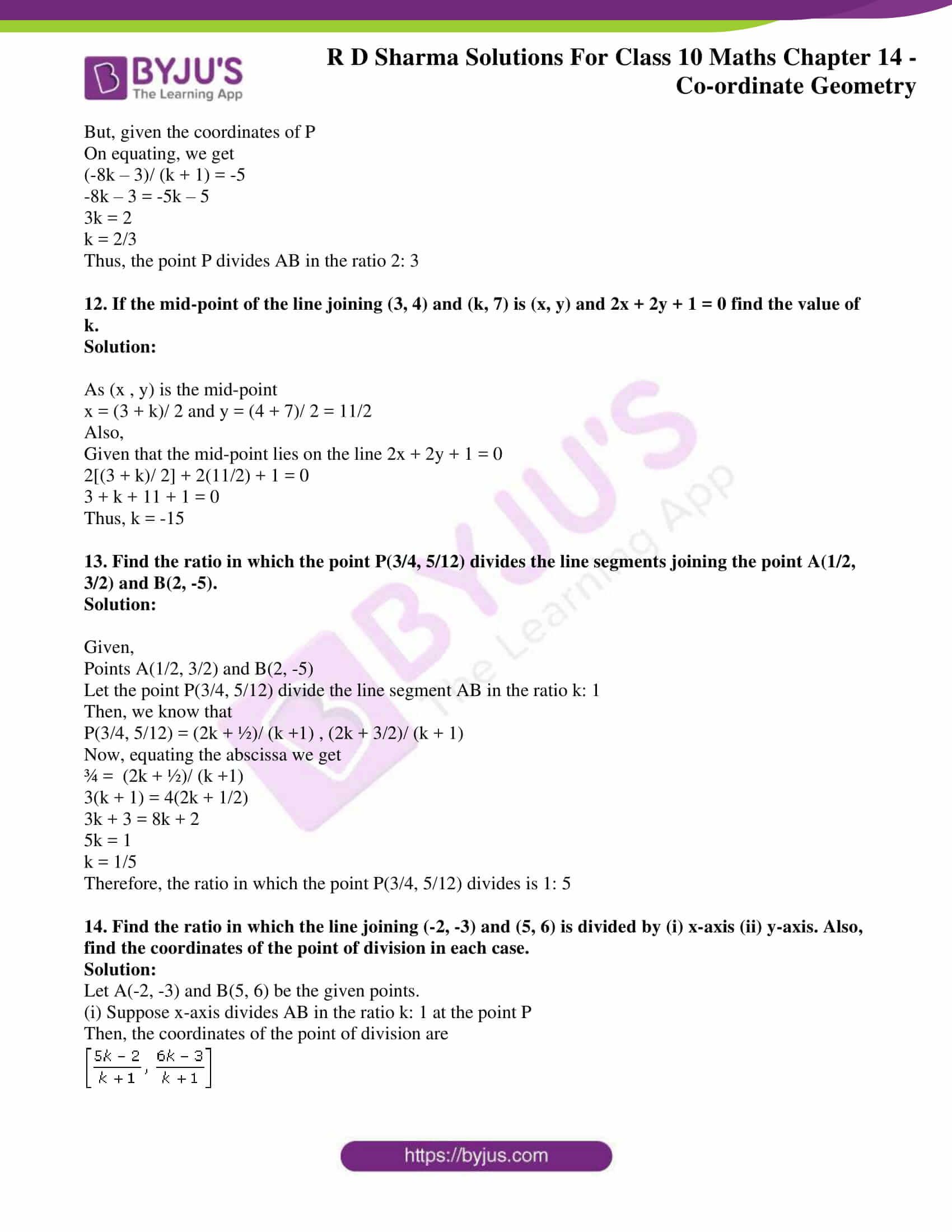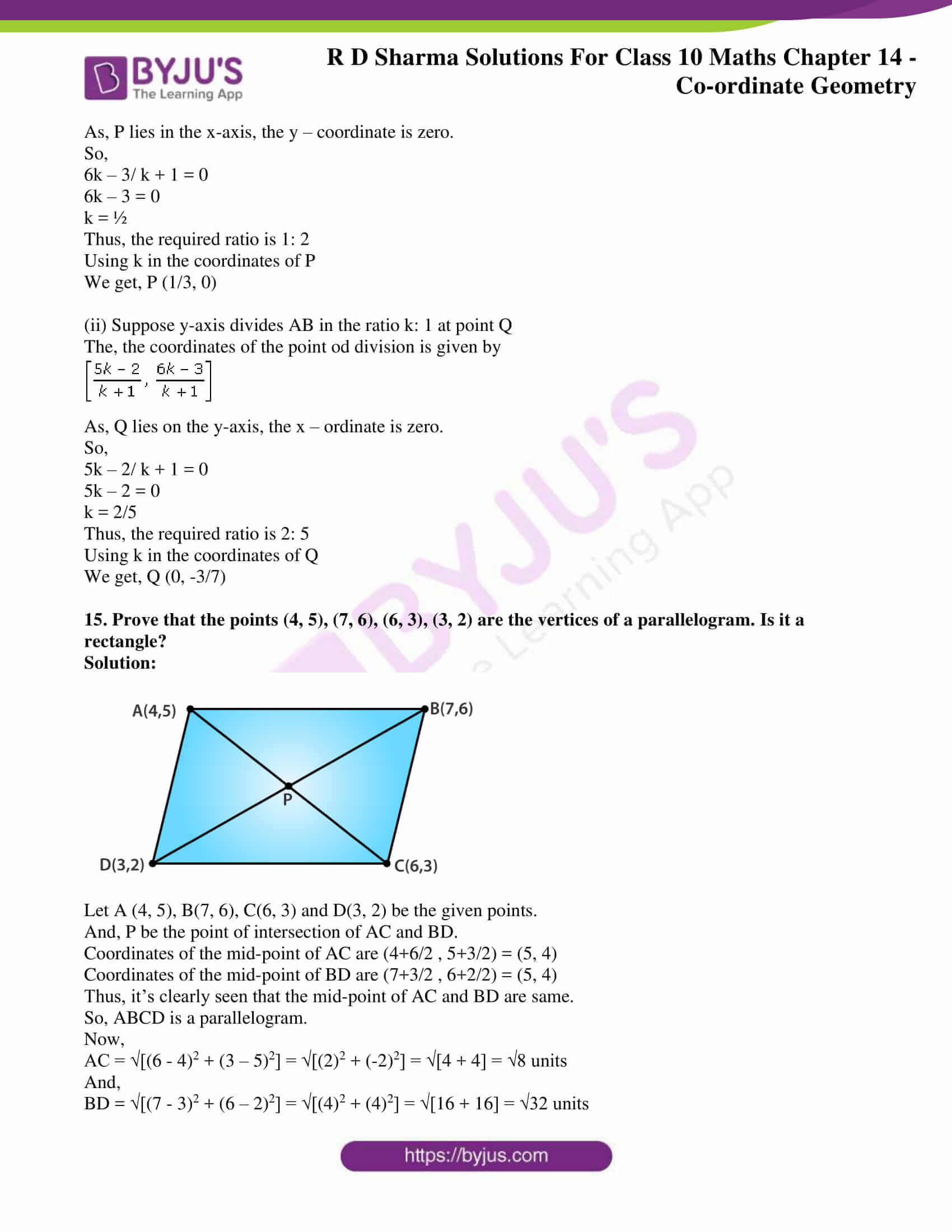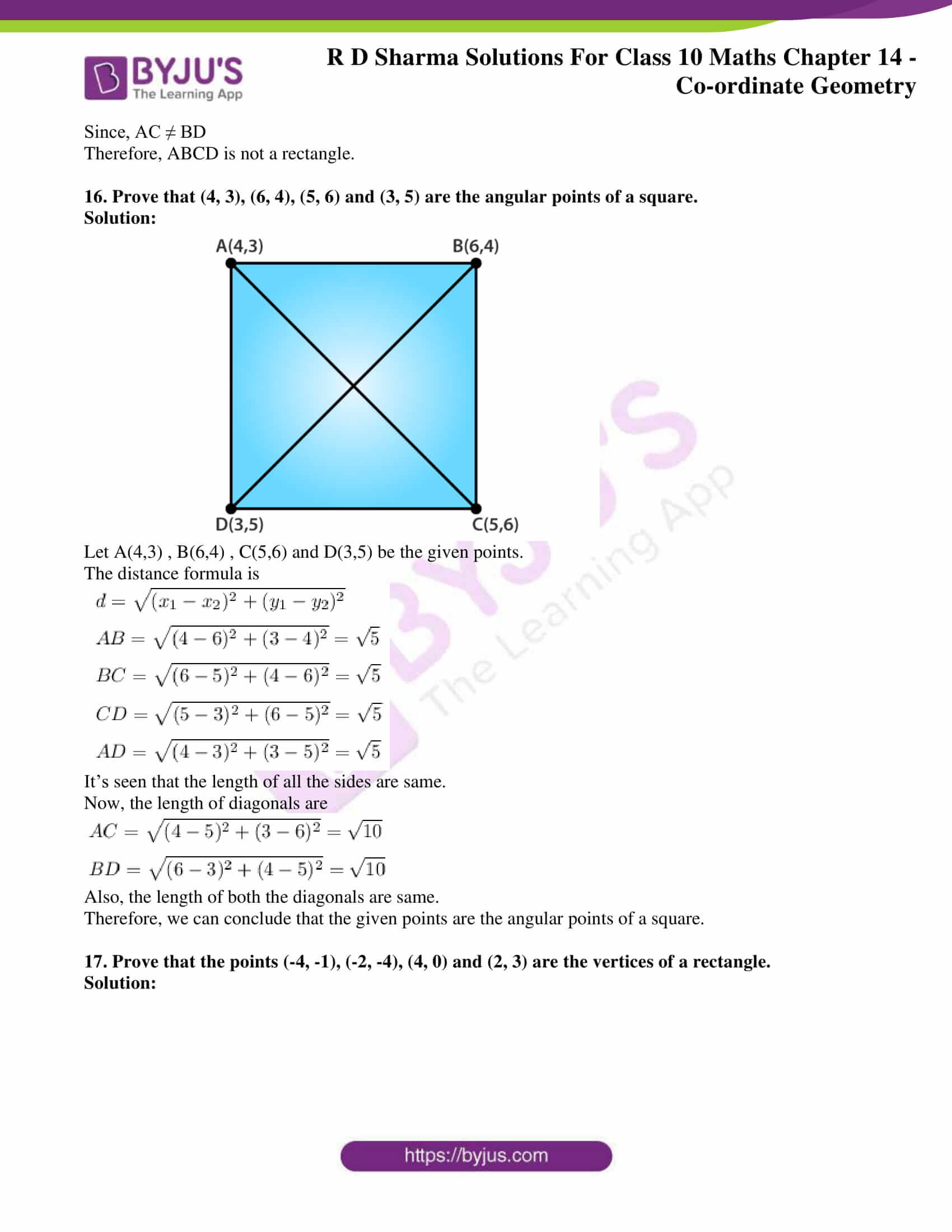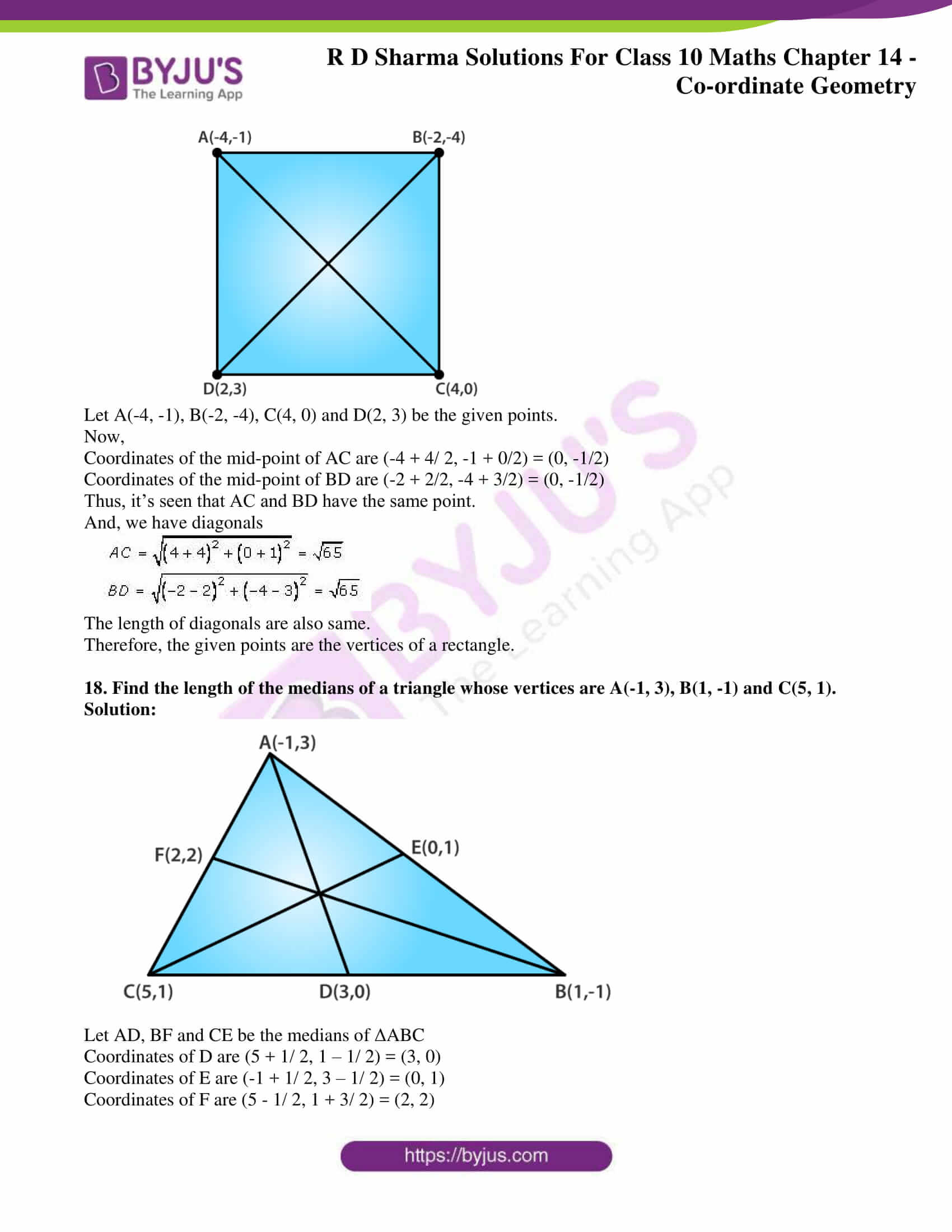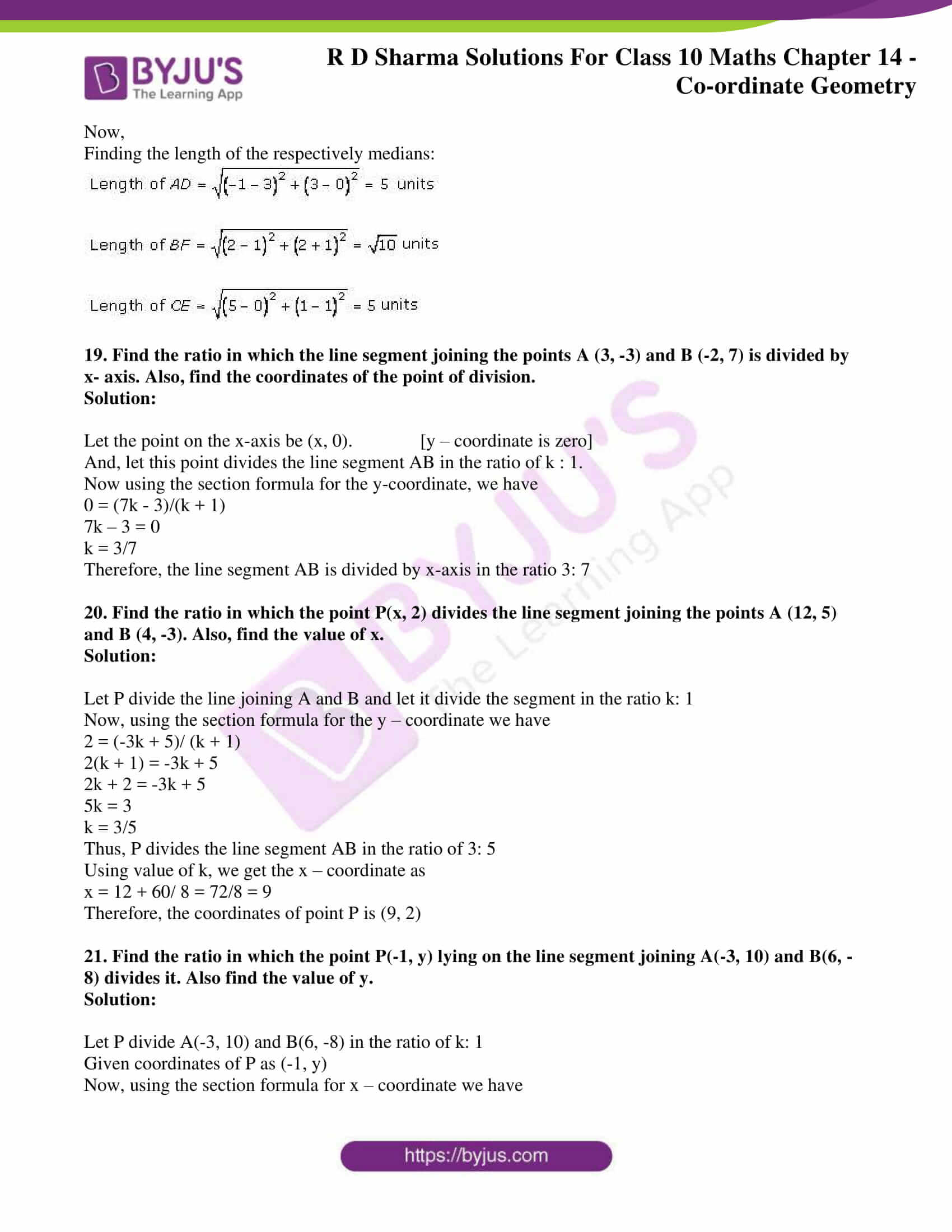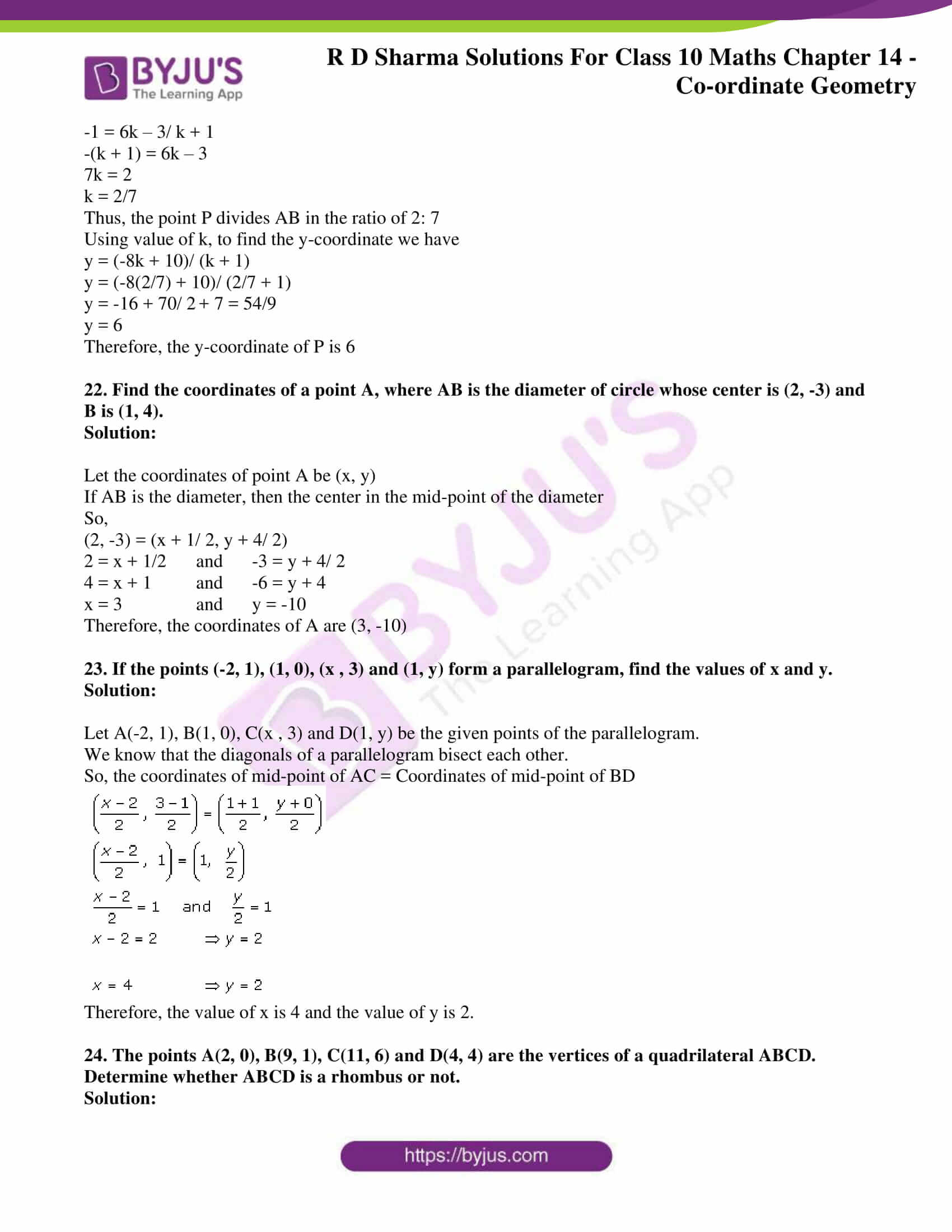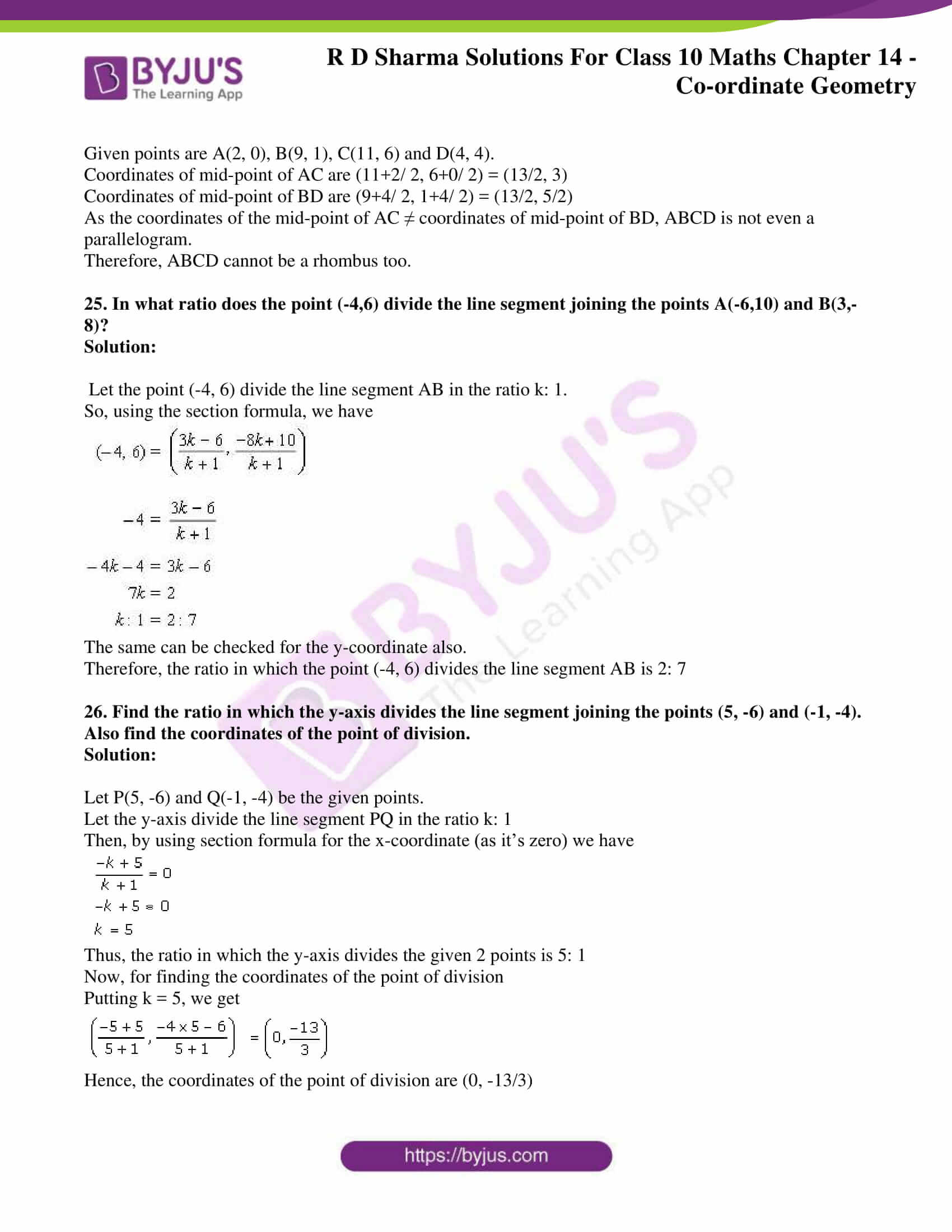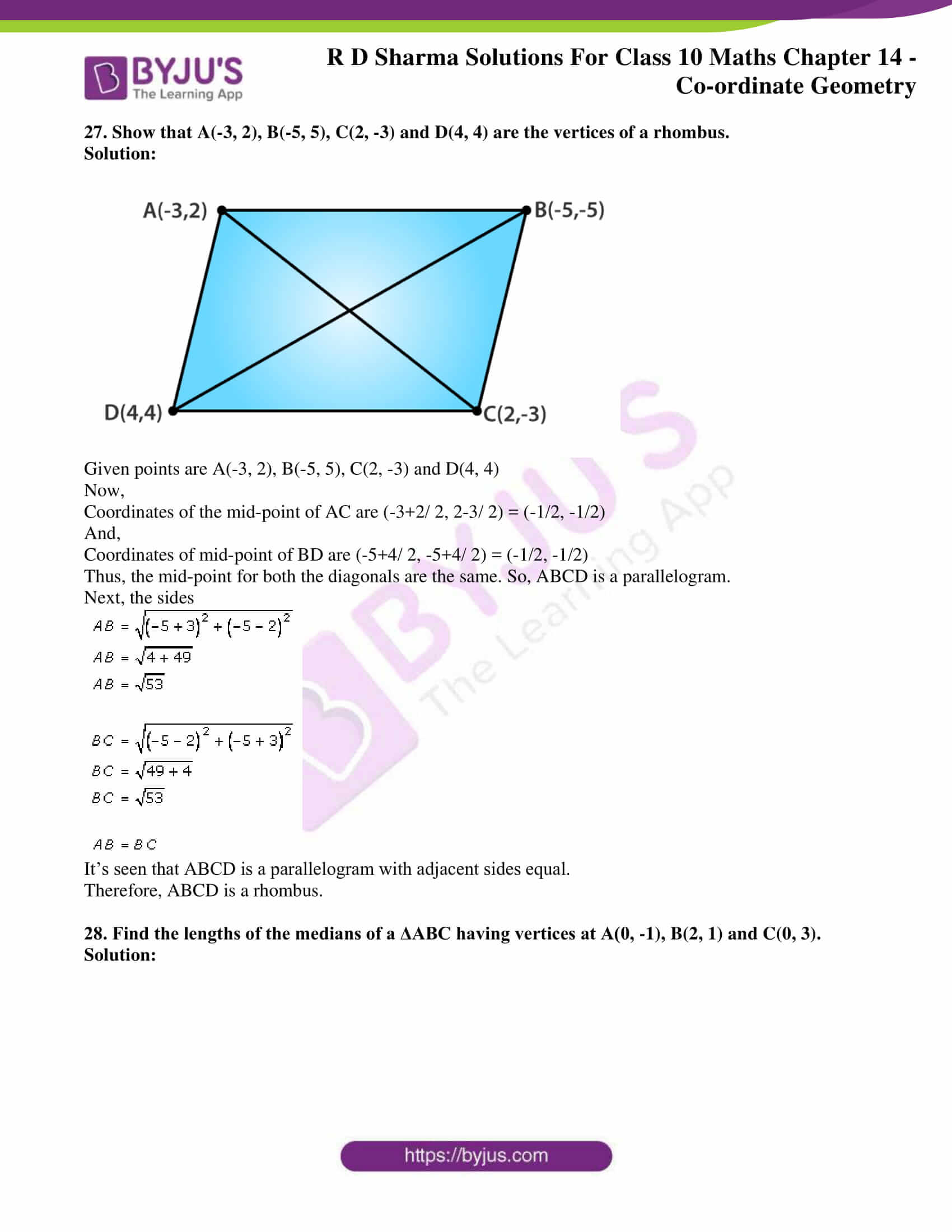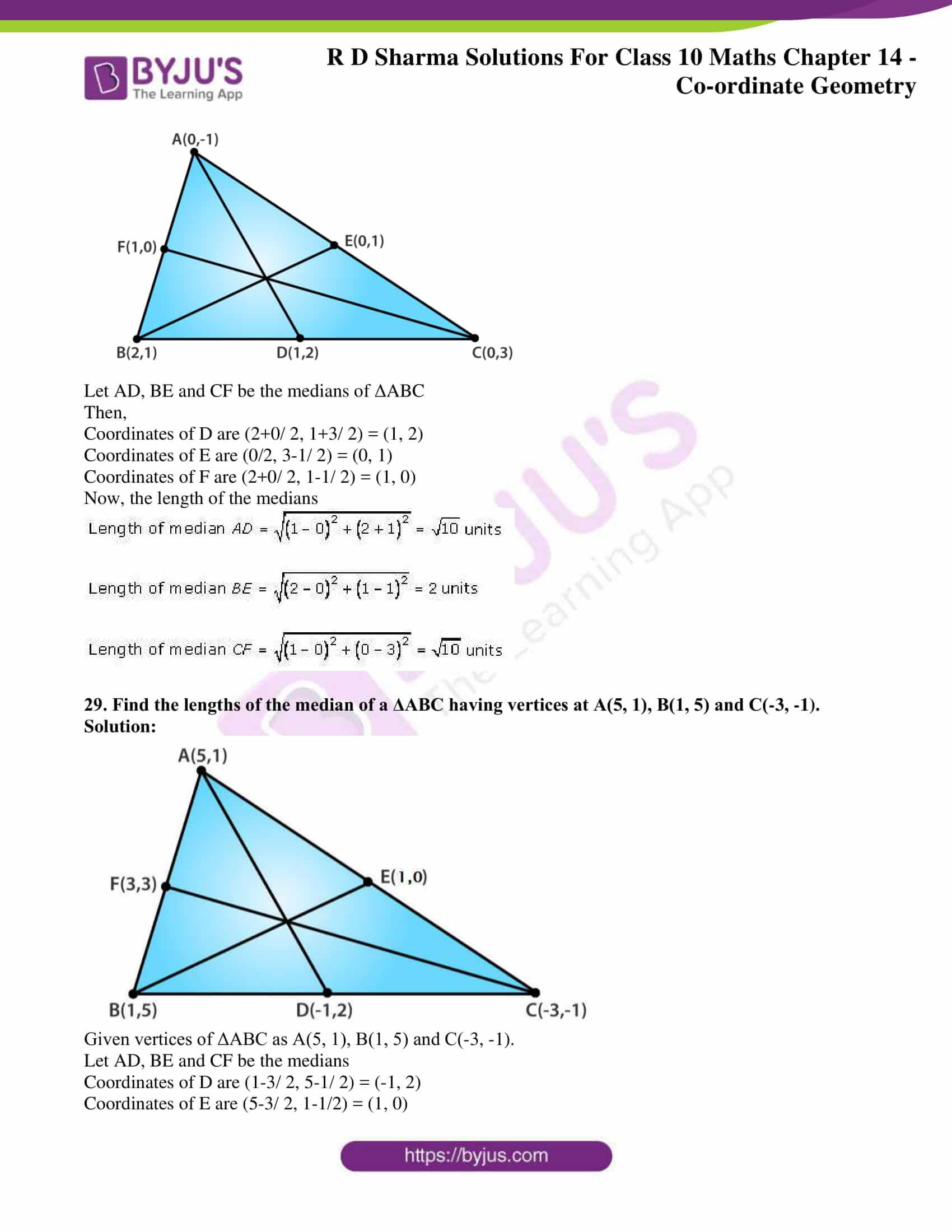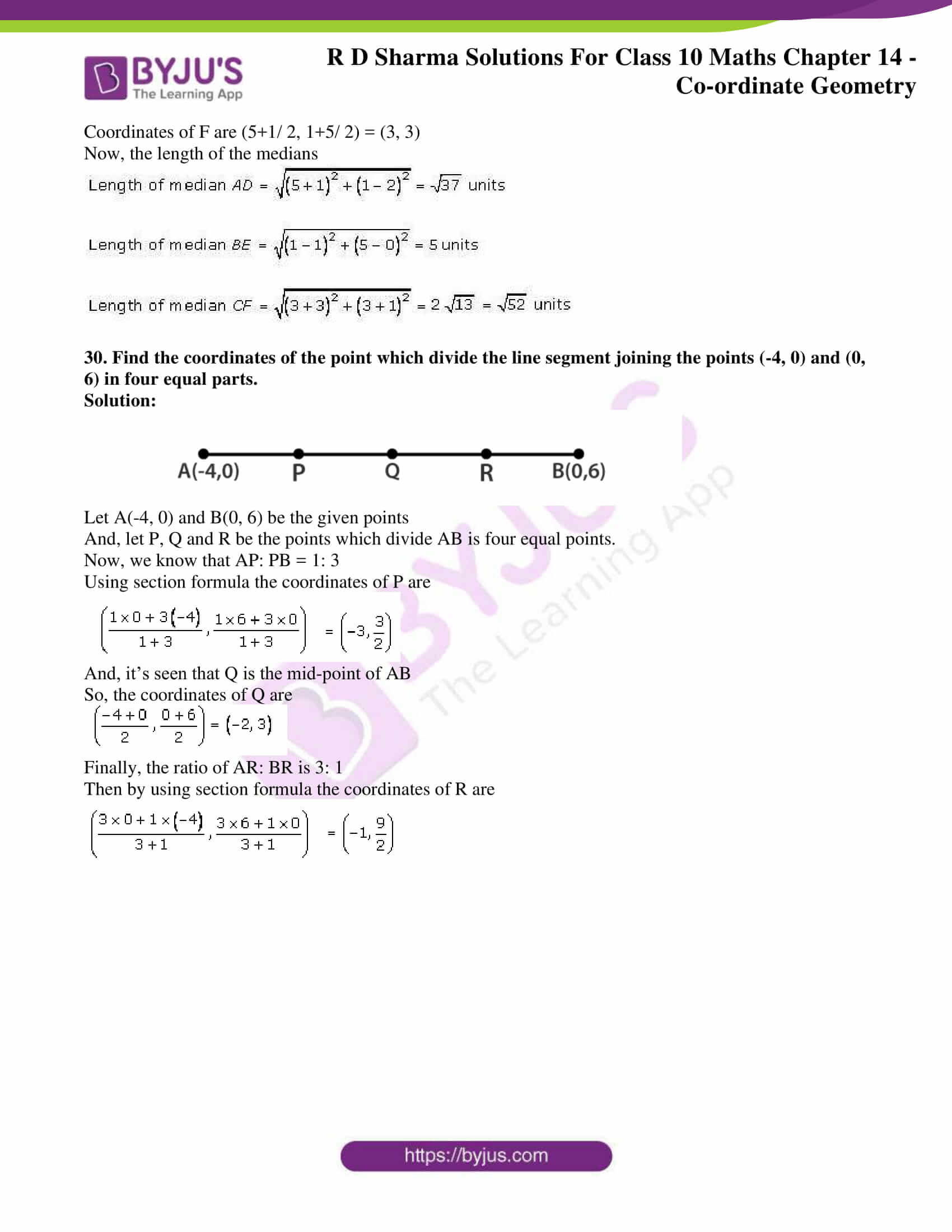### Access RD Sharma Solutions for Class 10 Maths Chapter 14 Co-ordinate Geometry Exercise 14.3

1. Find the coordinates of the point which divides the line segment joining (-1, 3) and (4, – 7) internally in the ratio 3: 4.

Solution:

Let P(x, y) be the required point.

By section formula, we know that the coordinates are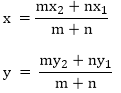Here,

x1 = – 1 y1 = 3

x2  = 4 y2 = -7

m: n = 3: 4

Then,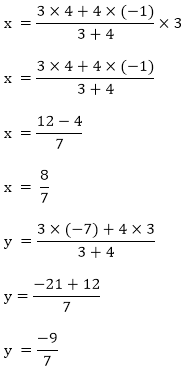Therefore, the coordinates of P are (8/7, – 9/7)

2. Find the points of trisection of the line segment joining the points:

(i) (5, – 6) and (-7, 5)

(ii) (3, – 2) and (-3, – 4)

(iii) (2, – 2) and (-7, 4)

Solution:

(i) Let P and Q be the point of trisection of AB such that AP = PQ = QB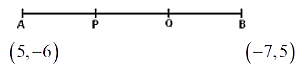So, P divides AB internally in the ratio of 1: 2, thereby applying section formula, the coordinates of P will be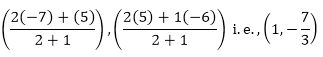Now, Q also divides AB internally in the ratio of 2:1 so their coordinates will be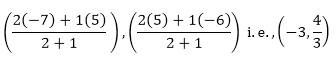(ii) Let P and Q be the points of trisection of AB such that AP = PQ = QB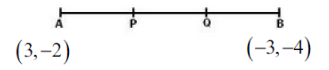As, P divides AB internally in the ratio of 1: 2. Hence by applying section formula, the coordinates of P are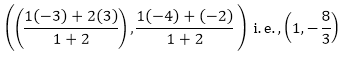Now, Q also divides as internally in the ratio of 2: 1

So, the coordinates of Q are given by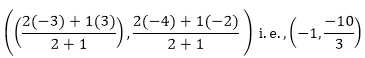(iii) Let P and Q be the points of trisection of AB such that AP = PQ = OQ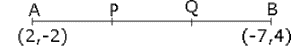As, P divides AB internally in the ratio 1:2. So, the coordinates of P, by applying the section formula, are given by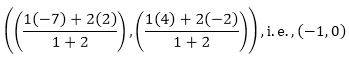Now. Q also divides AB internally in the ration 2: 1. And the coordinates of Q are given by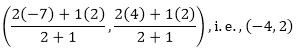3. Find the coordinates of the point where the diagonals of the parallelogram formed by joining the points (-2, -1), (1, 0), (4, 3) and (1, 2) meet.

Solution: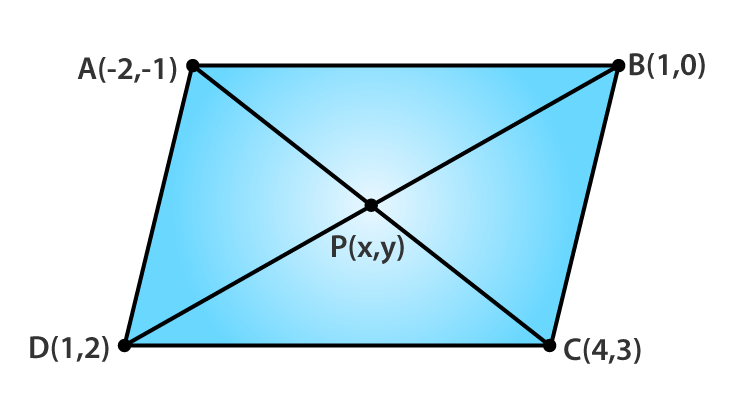Let A(-2, -1), B(1, 0), C(4, 3) and D(1, 2) be the given points.

Let P(x, y) be the point of intersection of the diagonals of the parallelogram formed by the given points.

We know that, diagonals of a parallelogram bisect each other.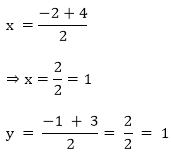Therefore, the coordinates of P are (1, 1)

4. Prove that the points (3, 2), (4, 0), (6, -3) and (5, -5) are the vertices of a parallelogram.

Solution: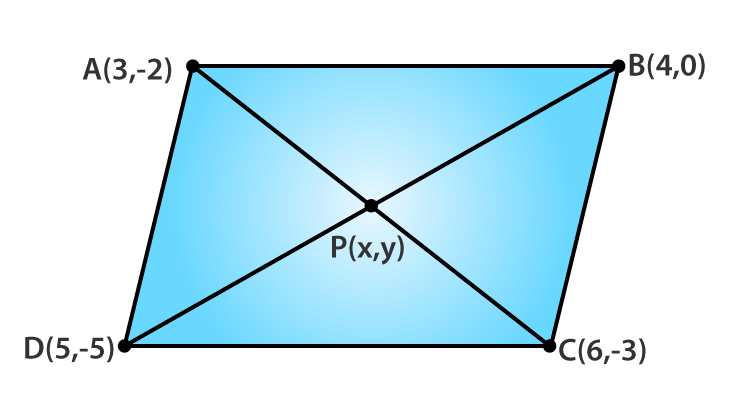Let A(3, -2), B(4, 0), C(6, -3) and D(5, -5)

Let P(x, y) be the point of intersection of diagonals AC and BD of ABCD.

The mid-point of AC is given by,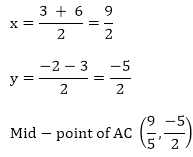Again, the mid-point of BD is given by,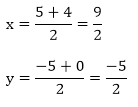Thus, we can conclude that diagonals AC and BD bisect each other.

And, we know that diagonals of a parallelogram bisect each other

Therefore, ABCD is a parallelogram.

5. If P(9a – 2, -b) divides the line segment joining A(3a + 1, -3) and B(8a, 5) in the ratio 3 : 1, find the values of a and b.

Solution:

Given that, P(9a – 2, -b) divides the line segment joining A(3a + 1, -3) and B(8a, 5) in the ratio 3:1

Then, by section formula

Coordinates of P are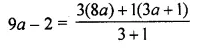And,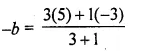Solving for a, we have

(9a – 2) x 4 = 24a + 3a + 1

36a – 8 = 27a + 1

9a = 9

a = 1

Now, solving for b, we have

4 x –b = 15 – 3

-4b = 12

b = -3

Therefore, the values of a and b are 1 and -3 respectively.

6. If (a, b) is the mid-point of the line segment joining the points A (10, -6), B(k, 4) and a – 2b = 18, find the value of k and the distance AB.

Solution:

As (a, b) is the mid-point of the line segment A(10, -6) and B(k, 4)

So,

(a, b) = (10 + k / 2, -6 + 4/ 2)

a = (10 + k)/ 2 and b = -1

2a = 10 + k

k = 2a – 10

Given, a – 2b = 18

Using b = -1 in the above relation we get,

a – 2(-1) = 18

a = 18 – 2 = 16

So,

k = 2(16) – 10 = 32 – 10 = 22

Thus,

AB = √[(22 – 10)2 + (4 + 6)2] = √[(12)2 + (10)2] = √[144 + 100] = 2√61 units

7. Find the ratio in which the point (2, y) divides the line segment joining the points A(-2, 2) and B(3, 7). Also find the value of y.

Solution:

Let the point P(2, y) divide the line segment joining the points A(-2, 2) and B(3, 7) in the ratio k: 1

Then, the coordinates of P are given by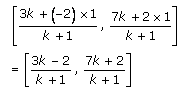And, given the coordinates of P are (2, y)

So,

2 = (3k – 2)/ (k + 1) and y = (7k + 2)/ (k + 1)

Solving for k, we get

2(k + 1) = (3k – 2)

2k + 2 = 3k – 2

k = 4

Using k to find y, we have

y = (7(4) + 2)/ (4 + 1)

= (28 + 2)/5

= 30/5

y = 6

Therefore, the ratio id 4: 1 and y = 6

8. If A(-1, 3), B(1, -1) and C(5, 1) are the vertices of a triangle ABC, find the length of median through A.

Solution: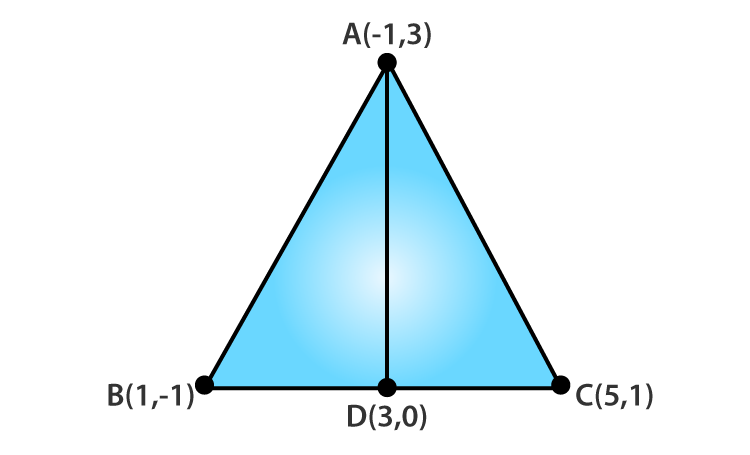Let AD be the median through A.

As, AD is the median, D is the mid-point of BC

So, the coordinates of D are (1 + 5/ 2, -1 + 1/ 2 ) = (3 , 0)

Therefore,

Length of median AD = √[(3 + 1)2 + (0 – 3)2] = √[(4)2 + (-3)2] = √[16 + 9] = √25 = 5 units

9. If the points P,Q(x, 7), R, S(6, y) in this order divide the line segment joining A(2, p) and B (7, 10) in 5 equal parts, find x, y and p.

Solution: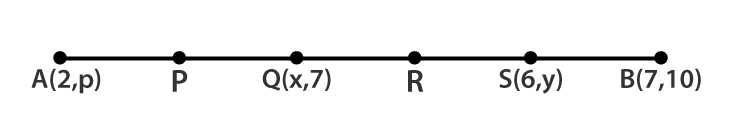From question, we have

AP = PQ = QR = RS = SB

So, Q is the mid-point of A and S

Then,

x = (2 + 6)/ 2 = 8/2 = 4

7 = (y + p)/ 2

y + p = 14 ….. (1)

Now, since S divides QB in the ratio 2: 1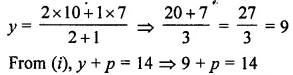So, p = 14 – 9 = 5

Therefore, x = 4, y = 9 and p = 5

10. If a vertex of a triangle be (1, 1) and the middle points of the sides through it be (-2, 3) and (5, 2) find the other vertices.

Solution:

Let A(1, 1) be the given vertex and D(-2, 3), E(5, 2) be the mid-points of AB and AC

Now, as D and E are the mid-points of AB and AC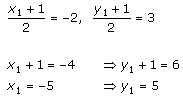So, the coordinates of B are (-5, 5)

Again,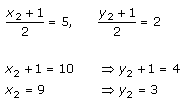So, the coordinates of C are (9, 3)

Therefore, the other vertices of the triangle are (-5, 5) and (9, 3).

11. (i) In what ratio is the segment joining the points (-2, -3) and (3, 7) divides by the y-axis? Also, find the coordinates of the point of division.

Solution:

Let P(-2, -3) and Q(9, 3) be the given points.

Suppose y-axis divides PQ in the ratio k: 1 at R(0, y)

So, the coordinates of R are given by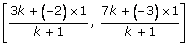Now, equating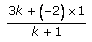= 0

3k – 2 = 0

k = 2/3

Therefore, the ratio is 2: 3

Putting k = 2/3 in the coordinates of R, we get

R (0, 1)

(ii) In what ratio is the line segment joining (-3, -1) and (-8, -9) divided at the point (-5, -21/5)?

Solution:

Let A(-3, -1) and B(-8, -9) be the given points.

And, let P be the point that divides AB in the ratio k: 1

So, the coordinates of P are given by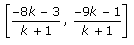But, given the coordinates of P

On equating, we get

(-8k – 3)/ (k + 1) = -5

-8k – 3 = -5k – 5

3k = 2

k = 2/3

Thus, the point P divides AB in the ratio 2: 3

12. If the mid-point of the line joining (3, 4) and (k, 7) is (x, y) and 2x + 2y + 1 = 0 find the value of k.

Solution:

As (x , y) is the mid-point

x = (3 + k)/ 2 and y = (4 + 7)/ 2 = 11/2

Also,

Given that the mid-point lies on the line 2x + 2y + 1 = 0

2[(3 + k)/ 2] + 2(11/2) + 1 = 0

3 + k + 11 + 1 = 0

Thus, k = -15

13. Find the ratio in which the point P(3/4, 5/12) divides the line segments joining the point A(1/2, 3/2) and B(2, -5).

Solution:

Given,

Points A(1/2, 3/2) and B(2, -5)

Let the point P(3/4, 5/12) divide the line segment AB in the ratio k: 1

Then, we know that

P(3/4, 5/12) = (2k + ½)/ (k +1) , (2k + 3/2)/ (k + 1)

Now, equating the abscissa we get

¾ = (2k + ½)/ (k +1)

3(k + 1) = 4(2k + 1/2)

3k + 3 = 8k + 2

5k = 1

k = 1/5

Therefore, the ratio in which the point P(3/4, 5/12) divides is 1: 5

14. Find the ratio in which the line joining (-2, -3) and (5, 6) is divided by (i) x-axis (ii) y-axis. Also, find the coordinates of the point of division in each case.

Solution:

Let A(-2, -3) and B(5, 6) be the given points.

(i) Suppose x-axis divides AB in the ratio k: 1 at the point P

Then, the coordinates of the point of division are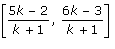As, P lies in the x-axis, the y – coordinate is zero.

So,

6k – 3/ k + 1 = 0

6k – 3 = 0

k = ½

Thus, the required ratio is 1: 2

Using k in the coordinates of P

We get, P (1/3, 0)

(ii) Suppose y-axis divides AB in the ratio k: 1 at point Q

The, the coordinates of the point od division is given byAs, Q lies on the y-axis, the x – ordinate is zero.

So,

5k – 2/ k + 1 = 0

5k – 2 = 0

k = 2/5

Thus, the required ratio is 2: 5

Using k in the coordinates of Q

We get, Q (0, -3/7)

15. Prove that the points (4, 5), (7, 6), (6, 3), (3, 2) are the vertices of a parallelogram. Is it a rectangle?

Solution: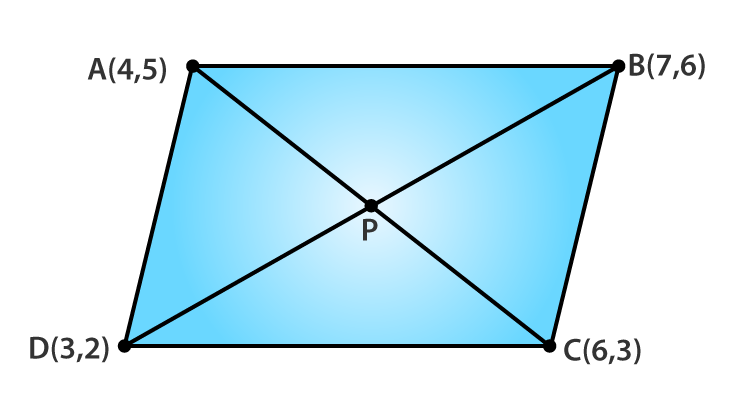Let A (4, 5), B(7, 6), C(6, 3) and D(3, 2) be the given points.

And, P be the point of intersection of AC and BD.

Coordinates of the mid-point of AC are (4+6/2 , 5+3/2) = (5, 4)

Coordinates of the mid-point of BD are (7+3/2 , 6+2/2) = (5, 4)

Thus, it’s clearly seen that the mid-point of AC and BD are same.

So, ABCD is a parallelogram.

Now,

AC = √[(6 – 4)2 + (3 – 5)2] = √[(2)2 + (-2)2] = √[4 + 4] = √8 units

And,

BD = √[(7 – 3)2 + (6 – 2)2] = √[(4)2 + (4)2] = √[16 + 16] = √32 units

Since, AC ≠ BD

Therefore, ABCD is not a rectangle.

16. Prove that (4, 3), (6, 4), (5, 6) and (3, 5) are the angular points of a square.

Solution: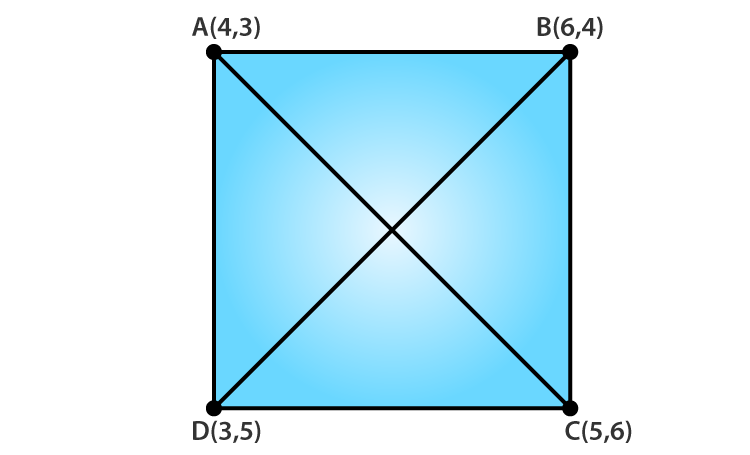Let A(4,3) , B(6,4) , C(5,6) and D(3,5) be the given points.

The distance formula is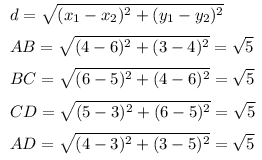It’s seen that the length of all the sides are same.

Now, the length of diagonals are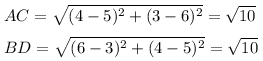Also, the length of both the diagonals are same.

Therefore, we can conclude that the given points are the angular points of a square.

17. Prove that the points (-4, -1), (-2, -4), (4, 0) and (2, 3) are the vertices of a rectangle.

Solution: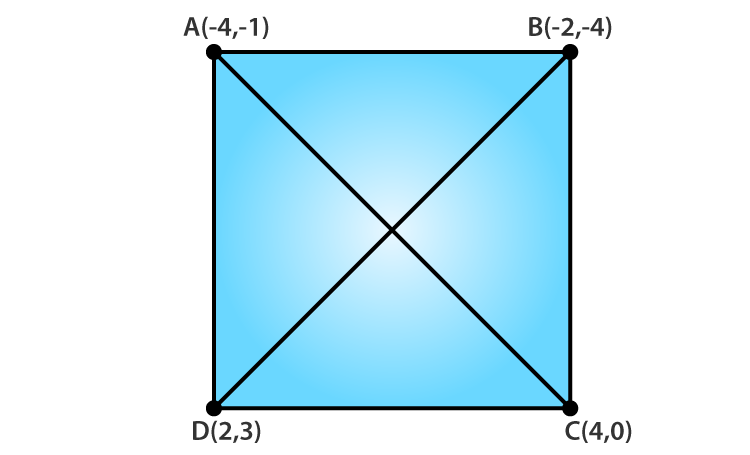Let A(-4, -1), B(-2, -4), C(4, 0) and D(2, 3) be the given points.

Now,

Coordinates of the mid-point of AC are (-4 + 4/ 2, -1 + 0/2) = (0, -1/2)

Coordinates of the mid-point of BD are (-2 + 2/2, -4 + 3/2) = (0, -1/2)

Thus, it’s seen that AC and BD have the same point.

And, we have diagonals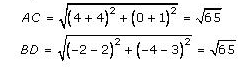The length of diagonals are also same.

Therefore, the given points are the vertices of a rectangle.

18. Find the length of the medians of a triangle whose vertices are A(-1, 3), B(1, -1) and C(5, 1).

Solution: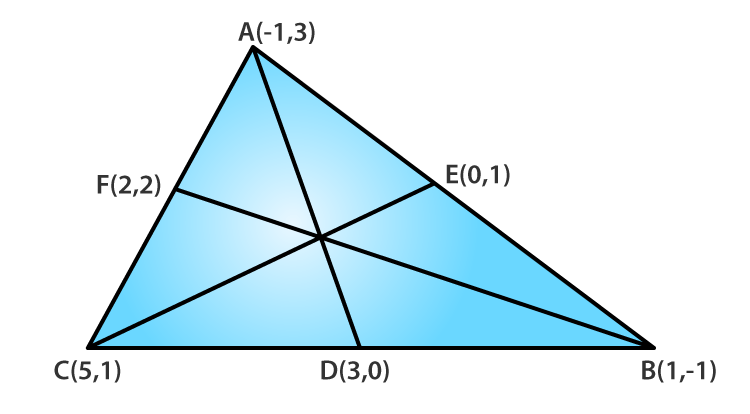Let AD, BF and CE be the medians of ΔABC

Coordinates of D are (5 + 1/ 2, 1 – 1/ 2) = (3, 0)

Coordinates of E are (-1 + 1/ 2, 3 – 1/ 2) = (0, 1)

Coordinates of F are (5 – 1/ 2, 1 + 3/ 2) = (2, 2)

Now,

Finding the length of the respectively medians: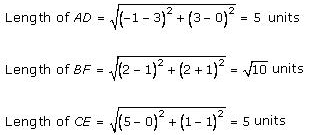19. Find the ratio in which the line segment joining the points A (3, -3) and B (-2, 7) is divided by x- axis. Also, find the coordinates of the point of division.

Solution:

Let the point on the x-axis be (x, 0). [y – coordinate is zero]

And, let this point divides the line segment AB in the ratio of k : 1.

Now using the section formula for the y-coordinate, we have

0 = (7k – 3)/(k + 1)

7k – 3 = 0

k = 3/7

Therefore, the line segment AB is divided by x-axis in the ratio 3: 7

20. Find the ratio in which the point P(x, 2) divides the line segment joining the points A (12, 5) and B (4, -3). Also, find the value of x.

Solution:

Let P divide the line joining A and B and let it divide the segment in the ratio k: 1

Now, using the section formula for the y – coordinate we have

2 = (-3k + 5)/ (k + 1)

2(k + 1) = -3k + 5

2k + 2 = -3k + 5

5k = 3

k = 3/5

Thus, P divides the line segment AB in the ratio of 3: 5

Using value of k, we get the x – coordinate as

x = 12 + 60/ 8 = 72/8 = 9

Therefore, the coordinates of point P is (9, 2)

21. Find the ratio in which the point P(-1, y) lying on the line segment joining A(-3, 10) and B(6, -8) divides it. Also find the value of y.

Solution:

Let P divide A(-3, 10) and B(6, -8) in the ratio of k: 1

Given coordinates of P as (-1, y)

Now, using the section formula for x – coordinate we have

-1 = 6k – 3/ k + 1

-(k + 1) = 6k – 3

7k = 2

k = 2/7

Thus, the point P divides AB in the ratio of 2: 7

Using value of k, to find the y-coordinate we have

y = (-8k + 10)/ (k + 1)

y = (-8(2/7) + 10)/ (2/7 + 1)

y = -16 + 70/ 2 + 7 = 54/9

y = 6

Therefore, the y-coordinate of P is 6

22. Find the coordinates of a point A, where AB is the diameter of circle whose center is (2, -3) and B is (1, 4).

Solution:

Let the coordinates of point A be (x, y)

If AB is the diameter, then the center in the mid-point of the diameter

So,

(2, -3) = (x + 1/ 2, y + 4/ 2)

2 = x + 1/2 and -3 = y + 4/ 2

4 = x + 1 and -6 = y + 4

x = 3 and y = -10

Therefore, the coordinates of A are (3, -10)

23. If the points (-2, 1), (1, 0), (x , 3) and (1, y) form a parallelogram, find the values of x and y.

Solution:

Let A(-2, 1), B(1, 0), C(x , 3) and D(1, y) be the given points of the parallelogram.

We know that the diagonals of a parallelogram bisect each other.

So, the coordinates of mid-point of AC = Coordinates of mid-point of BD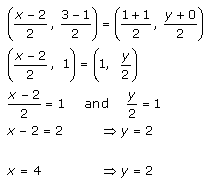Therefore, the value of x is 4 and the value of y is 2.

24. The points A(2, 0), B(9, 1), C(11, 6) and D(4, 4) are the vertices of a quadrilateral ABCD. Determine whether ABCD is a rhombus or not.

Solution:

Given points are A(2, 0), B(9, 1), C(11, 6) and D(4, 4).

Coordinates of mid-point of AC are (11+2/ 2, 6+0/ 2) = (13/2, 3)

Coordinates of mid-point of BD are (9+4/ 2, 1+4/ 2) = (13/2, 5/2)

As the coordinates of the mid-point of AC ≠ coordinates of mid-point of BD, ABCD is not even a parallelogram.

Therefore, ABCD cannot be a rhombus too.

25. In what ratio does the point (-4,6) divide the line segment joining the points A(-6,10) and B(3,-8)?

Solution:

Let the point (-4, 6) divide the line segment AB in the ratio k: 1.

So, using the section formula, we have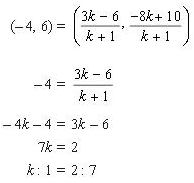The same can be checked for the y-coordinate also.

Therefore, the ratio in which the point (-4, 6) divides the line segment AB is 2: 7

26. Find the ratio in which the y-axis divides the line segment joining the points (5, -6) and (-1, -4). Also find the coordinates of the point of division.

Solution:

Let P(5, -6) and Q(-1, -4) be the given points.

Let the y-axis divide the line segment PQ in the ratio k: 1

Then, by using section formula for the x-coordinate (as it’s zero) we have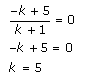Thus, the ratio in which the y-axis divides the given 2 points is 5: 1

Now, for finding the coordinates of the point of division

Putting k = 5, we get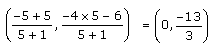Hence, the coordinates of the point of division are (0, -13/3)

27. Show that A(-3, 2), B(-5, 5), C(2, -3) and D(4, 4) are the vertices of a rhombus.

Solution: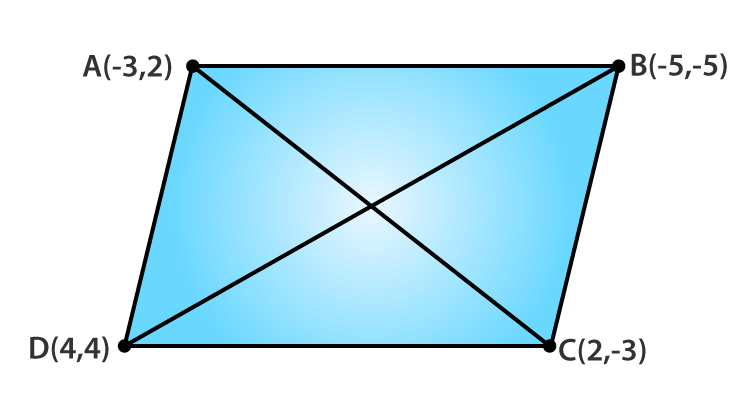Given points are A(-3, 2), B(-5, 5), C(2, -3) and D(4, 4)

Now,

Coordinates of the mid-point of AC are (-3+2/ 2, 2-3/ 2) = (-1/2, -1/2)

And,

Coordinates of mid-point of BD are (-5+4/ 2, -5+4/ 2) = (-1/2, -1/2)

Thus, the mid-point for both the diagonals are the same. So, ABCD is a parallelogram.

Next, the sides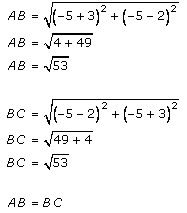It’s seen that ABCD is a parallelogram with adjacent sides equal.

Therefore, ABCD is a rhombus.

28. Find the lengths of the medians of a ΔABC having vertices at A(0, -1), B(2, 1) and C(0, 3).

Solution: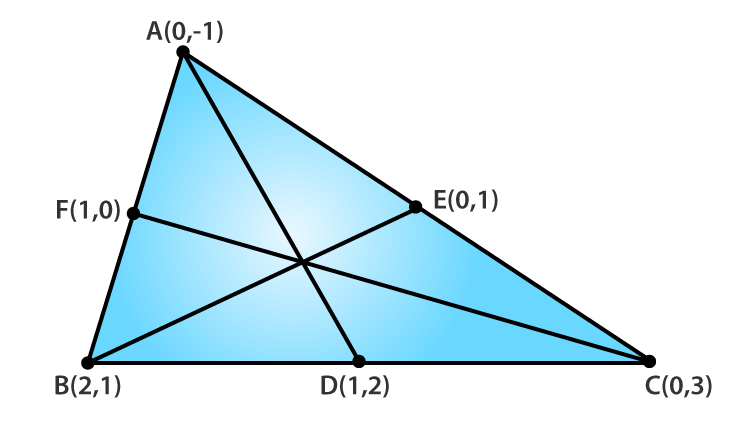Let AD, BE and CF be the medians of ΔABC

Then,

Coordinates of D are (2+0/ 2, 1+3/ 2) = (1, 2)

Coordinates of E are (0/2, 3-1/ 2) = (0, 1)

Coordinates of F are (2+0/ 2, 1-1/ 2) = (1, 0)

Now, the length of the medians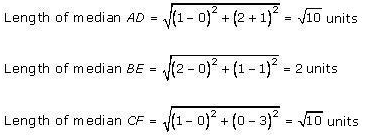29. Find the lengths of the median of a ΔABC having vertices at A(5, 1), B(1, 5) and C(-3, -1).

Solution: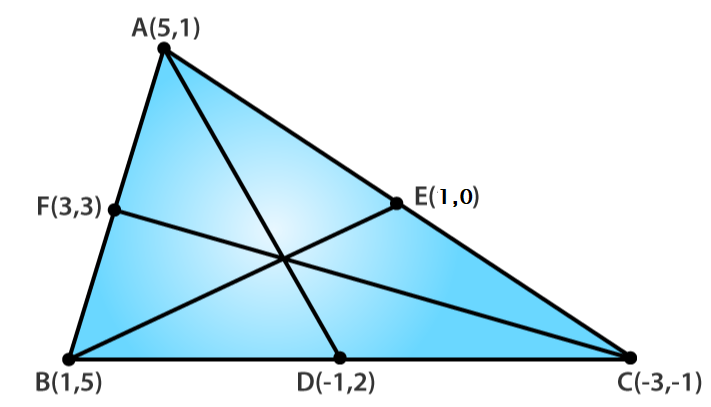Given vertices of ΔABC as A(5, 1), B(1, 5) and C(-3, -1).

Let AD, BE and CF be the medians

Coordinates of D are (1-3/ 2, 5-1/ 2) = (-1, 2)

Coordinates of E are (5-3/ 2, 1-1/2) = (1, 0)

Coordinates of F are (5+1/ 2, 1+5/ 2) = (3, 3)

Now, the length of the medians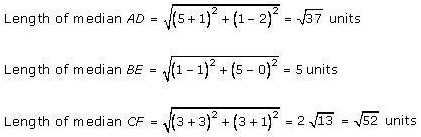30. Find the coordinates of the point which divide the line segment joining the points (-4, 0) and (0, 6) in four equal parts.

Solution:Let A(-4, 0) and B(0, 6) be the given points

And, let P, Q and R be the points which divide AB is four equal points.

Now, we know that AP: PB = 1: 3

Using section formula the coordinates of P are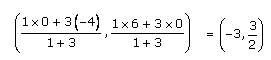And, it’s seen that Q is the mid-point of AB

So, the coordinates of Q are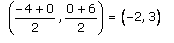Finally, the ratio of AR: BR is 3: 1

Then by using section formula the coordinates of R are